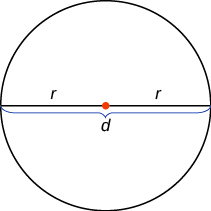## Key Concepts

• Convert a Fraction to a Decimal To convert a fraction to a decimal, divide the numerator of the fraction by the denominator of the fraction.
• Properties of Circles$r$ is the length of the radius

$d$ is the length of the diameter

The circumference is $2\pi r$ , $C=2\pi r$

The area is $\pi {r}^{2}$ , $A=\pi {r}^{2}$

## Glossary

circumference of a circle
The distance around a circle is called its circumference.
diameter of a circle
A diameter of a circle is a line segment that passes through a circle’s center connecting two points on the circle.